# Model:Morrell SSBM

## Morrell single-stage ball mill Model

This is a secondary and tertiary cone crusher, plus ball mill model that estimates the specific energy consumption using the equations of Morrell (GMSG, 2016). The secondary crusher operates in open circuit and the tertiary crusher is in closed circuit with a screen.

### Testwork Required

• The SMC™ test, stored in the "DWT" testwork table (SMC is a Drop Weight Test).
• Bond ball mill work index. The method requires all the elements of the ball mill work index test to be filled in:
• Ball mill Wi test closing screen size, umclosing, µm
• Ball mill Wi test product size, P80, µm
• Ball mill Wi test feed size, F80, µm
• Ball mill Wi grams per revolution at test conclusion, gpr

### Required parameters

• F80, µm is the 80% passing size of the fresh feed to the circuit, ie, primary crusher product (expected to be a Bond-compatible size distribution).
• Pc80, µm is the 80% passing size of the secondary crusher product (expected to be a Bond-compatible size distribution).
• T80, µm is the 80% passing size of the tertiary crusher screen undersize, ie. ball mill circuit feed (expected to be a Bond-compatible size distribution).
• P80, µm is the 80% passing size of the circuit product (expected to be a Bond-compatible size distribution).
• Availability, expressed as a decimal (0.90 = 90% availability) is used to convert t/h to t/d.

### Optional parameters

• Description and Comment are optional text fields
• Maximum t/h limit is a t/h throughput above which a warning message is displayed (but does not actually limit the throughput)
• Edust.collectn is the specific energy consumed by dust collection (default is 0.5 kWh/t)
• Conveyor lift height, used to estimate the specific energy consumption of the conveyors (default is 15 m)

### Formulae

The method is superficially similar to Bond (among other models), but with a variable exponent on size defined by: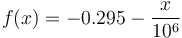$f(x) = - 0.295 - \frac{x}{10^6}$

Where x is the particle 80% passing size in µm.

Secondary crushing circuit, assumes secondary crusher operates in closed circuit with a screen: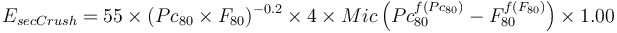$E_{secCrush} = 55 \times (Pc_{80} \times F_{80})^{-0.2} \times 4 \times Mic \left( Pc_{80}^{f(Pc_{80})} - F_{80}^{f(F_{80})} \right) \times 1.00$GMSG, 2016

Tertiary crusher, assumes operating in closed circuit with a screen: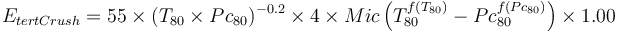$E_{tertCrush} = 55 \times (T_{80} \times Pc_{80})^{-0.2} \times 4 \times Mic \left( T_{80}^{f(T_{80})} - Pc_{80}^{f(Pc_{80})} \right) \times 1.00$GMSG, 2016

Ball mill circuit: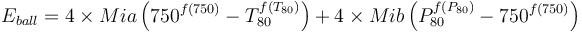$E_{ball} = 4 \times Mia \left( 750^{f(750)} - T_{80}^{f(T_{80})} \right) + 4 \times Mib \left( P_{80}^{f(P_{80})} - 750^{f(750)} \right)$GMSG, 2016

### Discussion

• This model requires that the product of the secondary and tertiary crushers be coarser than 750 µm. This is reasonable as no crushing circuit operates anywhere near that size range.
• There is a minor correction required for open circuit secondary crushing (the 1.0 factor becomes 1.19). In theory, open circuit operation would change the particle size distribution of the secondary crusher product affecting the load on the tertiary crusher. The change in secondary crusher specific energy consumption should be, for all practical purposes, completely offset by a change in the tertiary crusher specific energy consumption.
• The estimation of dust collection is based on Doll, 2015 where Edust = 0.5 kWh/t.
• The estimation of conveyor specific energy is based on Doll, 2015: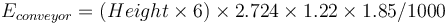$E_{conveyor} = (Height \times 6) \times 2.724 \times 1.22 \times 1.85 / 1000$